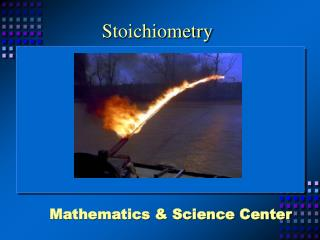DownloadDownload PresentationStoichiometry

# Stoichiometry

Télécharger la présentation## Stoichiometry

- - - - - - - - - - - - - - - - - - - - - - - - - - - E N D - - - - - - - - - - - - - - - - - - - - - - - - - - -
##### Presentation Transcript

1. Stoichiometry Mathematics & Science Center

3. Mole A Counting Number Equal To 6.02 X 1023 individual units. A mass in grams equal to the atomic mass or the formula mass of a substance.

4. Set the ratio of the last two cells in the given column equal to the ratio of the last two cells in the column containing ‘x’. All quantities except ‘x’ should have a label. 4 Solve for ‘x’ and express the final answer with the correct label. 5 Write a Balanced Equation. State the Problem Above the Reactants and Products in the Equation Add another row of cells. Restate the moles of the given and unknown quantities in the appropriate units. 2 Add an additional row of cells. State the given and unknown quantities. 3 Create a basic equation table from the balanced equation. 1 C3H8 + 5O2 → 4 H2O + 3 CO2 50 moles ? mL

5. Exercise 1: Spacecraft Air 2LiOH + CO2Li2CO3 + H2O

6. ? grams 1400. g 2LiOH + CO2 Li2CO3 + H2O 2 moles 1 mole 47.8 g 44.0 g X 1400. g 44.0 g CO2 1400. g CO2 47.8 g LiOH X =

7. 44.0 g CO2 1400. g CO2 47.8 g LiOH X = (47.8g LiOH) (1400. g CO2) X = _______________________________________________ 44.0 g CO2 1520g LiOH X =

8. Exercise 2: Combination Reaction 2 H2 + O2 2H2O

9. ? molecules 550. g 2 H2 + O2 2H2O 2 moles 1 mole 6.02 x 1023 36.0 g molecules x 550. g 36.0 g H2O = 6.02 x 1023 molecules O2 550. g H2O x X = 9.20 x 1024 molecules O2

10. Exercise 3: Flamethrower 12.9 g ?g ? molecules ? mL C3H8 + 5O2 3CO2 + 4H2O 46.9 g O2 5.29 x 1023 molecules 21.1 mL H2O

11. Limiting Reactant? Exercise 4: Combustion Reaction C + O2 -----> CO2 A charcoal briquette weighing 45 g is placed in a jar containing 115. g of oxygen. The briquette is lit and a lid is placed on the jar. How many grams of CO2 can be produced by this combustion reaction?

12. 45g 115 g ?g C + O2 ----> CO2 1mole 1mole 1mole 12.0 g 32.0 g 44.0 g 45.0 g 115 g X X = 165 g CO2 X = 158 g CO2 Oxygen is the limiting reagent!

13. Online Practice Electronic Flashcard http://garnet.acns.fsu.edu/~rlight/stoich/

14. What is stoichiometry?